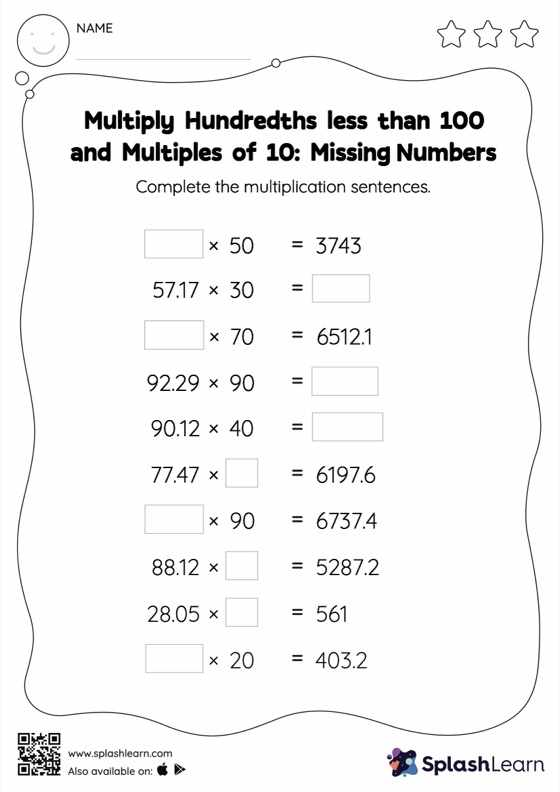# Multiply Hundredths less than 100 and Multiples of 10: Missing Numbers Worksheet

Home > Multiply Hundredths less than 100 and Multiples of 10: Missing NumbersHelp your little one develop a knack for math with this multiply hundredths less than 100 and multiples of 10 worksheet. Students find the missing number in decimal multiplication in multiply hundredths less than 100 and multiples of 10 worksheet. To do this, they apply the understanding that when multiplying a decimal by a number that ends in zeros, the decimal point will be moved to the right by the same number of places as the multiplier's number of zeros.